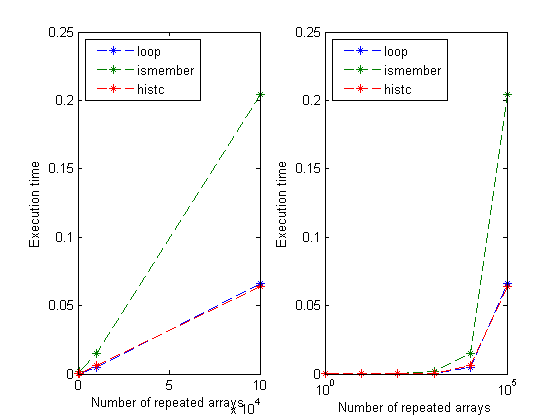## Loren on the Art of MATLABTurn ideas into MATLAB

Note

Loren on the Art of MATLAB has been archived and will not be updated.

# Inverse Mapping from Values to Indices

I am aware of frequent customer requests for replacing multiple values in an array. And the result should be compact code and execute quickly, right?! It came up again on a recent thread on the MATLAB newsgroup so it seemed timely to write an article on this topic for the archives. In this recent thread, the question of timing came up - which method is fastest? Your mileage may vary and it would be interesting to compare results, especially if yours are qualitatively different than those run from my laptop. You can post your results in the comments below.

### Contents

Tidy up to start.

clear
format short g

### Sample Input and Results

Supppose I have an array a containing values that I expect to find in another array, Minit.

a = [1 3 5 7 8]
Minit = [1 3 5; ...
3 5 7; ...
3 7 8]
a =
1     3     5     7     8
Minit =
1     3     5
3     5     7
3     7     8


I would like to see a replacement matrix for Minit in which the values are replaced with their indices in the list a.

Mwanted = [1 2 3; ...
2 3 4; ...
2 4 5]
Mwanted =
1     2     3
2     3     4
2     4     5


### Algorithms

There are at least three ways to accomplish the task, one, a brute force loop, and two that require knowledge of other very useful MATLAB functions, histc and ismember.

Let's first look at the for-loop solution.

type loopFR
function M1 = loopFR(M,A)
% loopFR replaces M with its indices from A.

% First set up the inverse mapping array.  Initialize it to zero, and the
% size is the maximum number the array.  Note that I get away with this
% here because all of my numbers in both A and M are positive integers.
imap = zeros(1,max(A));  % inverse mapping array
% Now fill the inverse map, but placing in the location specified in each
% element of A, the index of that element in A.
% If A = [1 5 4], then imap = [1 0 0 3 2].
imap(A) = 1:length(A);
M1 = M;
for k = 1:numel(M)
M1(k) = imap(M(k));
end


Here, I set up imap, an array that allows me to do the inverse mapping from values in a back to indices in a.

And check that loopFR gets the right result.

isequal(loopFR(Minit,a), Mwanted)
ans =
1


Now let's check both ismember and histc before spending effort timing the results.

[mem, mem] = ismember(Minit,a);
[his, his] = histc(Minit,a);
isequal(Mwanted, mem, his)
ans =
1


### Time the Algorithms

N = [1 10 100 1000 10000 100000];  % repetitions to test
t = zeros(length(N),3); % set up the timing collection array
for n = 1:length(N)
% Make a larger matrix to search.
% loopFR
M = repmat(Minit,N(n),1);
tic
M1 = loopFR(M,a);
t(n,1) = toc;
% ismember
tic
[M2,M2] = ismember(M,a);
t(n,2) = toc;
% histc
tic
[M3,M3] = histc(M,a);
t(n,3) = toc;
if ~isequal(M1,M2,M3)
disp('oops')
end
end

### Timing Results

disp('       Counter     numel(M)     loop       ismember     histc')
disp([N' 9*N' t]);
subplot(1,2,1)
plot(N', t, '--*')
xlabel('Number of repeated arrays')
ylabel('Execution time')
legend({'loop','ismember','histc'},'Location', 'NorthWest')
subplot(1,2,2)
semilogx(N', t, '--*')
xlabel('Number of repeated arrays')
ylabel('Execution time')
legend({'loop','ismember','histc'},'Location', 'NorthWest')
       Counter     numel(M)     loop       ismember     histc
1            9  4.5537e-005   0.00012627  1.2292e-005
10           90  2.8775e-005  8.8838e-005  1.3689e-005
100          900  6.6489e-005   0.00022042  5.8108e-005
1000         9000   0.00046542    0.0017497   0.00051291
10000        90000     0.004678     0.015192    0.0059801
1e+005       9e+005     0.065959      0.20439     0.063979